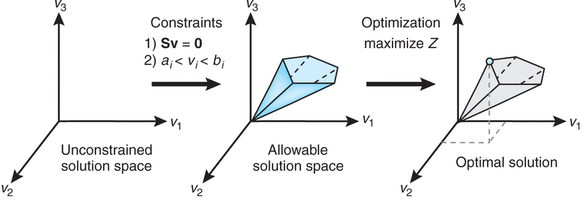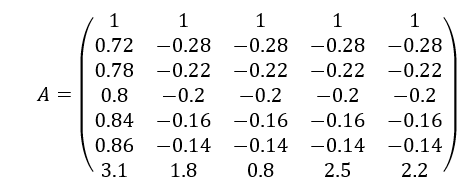Digital analysts are constantly asked to answer the question: Which are the most important marketing channels our company should focus on as we develop a digital marketing model?

Determining the true importance of each marketing channel is called attribution modelling.

Attribution modelling has seen a lot of interest in measuring relative importance in recent years and it can determine the budget allocated for each channel.

It is important to note that giving all the credit to the last channel is not fair because a customer usually uses multiple channels before a purchase.

It is not always the last channel that convinces customers to make a purchase: the previous channels also play a role.

That’s why we argue that first off we need to solve attribution problems before making a hasty or immediate decision after looking at the last channel report that can often be misleading.

A better approach is to employ some other techniques, such as Google data-driven attribution, survival analysis, relative importance and so on.

#### Attribution modelling and budget allocation for you digital marketing model

In order to allocate budget for marketing channels for your digital marketing model, marketers often leverage the concept of relative importance to determine the relative contribution of each channel. Variable importance in regression refers to the quantification of an individual regressor’s contribution to multiple regression models.

Common models to analyse relative importance include regression models, such as the Shapley value regression, and pmvd (a newly proposed model by Fledman).

In addition to multivariate linear regression, some other models such as random forest have received a lot of attention recently for assessment of variable importance.

Despite the popularity of relative importance, these models cannot cover all the complexities we encounter in real life.

Often, marketers have to include additional constraints necessitated by the market or an organisation’s strategies.

In this respect, we can use linear programming to allocate budget to each marketing activity and at the same time cover all the constraints.

#### Using Linear Programming for budget allocation

In this article, using an example and linear programming, we explain how to allocate budget to marketing channels.

In order to solve an optimization problem using linear programming, we need a set of linear constraints. In mathematical terms, linear programming is a method in the field of operation management whose requirements are represented by linear relationships explained as follows:

• Maximise the objective function (such as maximum profit or lowest cost)

\$\$C^T * x \$\$

• Subject to set of linear constraints

\$\$Ax le b\$\$

• ( x_i ge 0) for each (i)

Here, (c_i ) represents Return-On-Investment (ROI) and (x) represents the amount invested in each asset and the set of constraints are presented in (A) matrix. Linear programming is solved with the simplex algorithm.As an example, let’s say we have five marketing channels as follows:

Constraints need to be expressed in a common format as linear inequalities, i.e. for the (j) constraint:

\$\$a_1 * x_1 + a_2 * x_2 + a_3 * x_3 + a_4 * x_4 + a_5 * x_5 le b_j \$\$

The set of linear constraints is listed as follows:

• First constraint: The total budget is \$100,000.

\$\$x_1 + x_2 + x_3 +x_4 + x_5 le 100,000\$\$

• Second constraint: in this example we stipulate that the TV advertisement budget must not exceed 58% of the whole budget, so

\$\$x_1 le 0.58 (x_1 + x_2 + x_3 + x_4 + x_5)\$\$

That is expressed as:

\$\$0.42 x_1 -0.58 x_2 – 0.58 x_3 -0.58 x_4 – 0.58 x_5 le 0\$\$

• Third constraint: sum of Google / CPC and TV advertisement budget must not exceed 80% of the whole budget

\$\$(x_1 + x_2) le 0.8 (x_1 + x_2 + x_3 + x_4 + x_5)\$\$

\$\$0.2 x_1 + 0.2 x_2 – 0.8 x_3 – 0.8 x_4 – 0.8x_5 le 0\$\$

• Fourth constraint: Some of Twitter / CPC, Google CPC and Google Display budget must not exceed 30% of the whole budget

\$\$(x_2 + x_3 +x_5) le 0.3 (x_1 + x_2 + x_3 + x_4 + x_5)\$\$

\$\$-0.3 x_1 + 0.7 x_2 + 0.7 x_3 -0.3 x_4 – 0.7 x_5 le 0\$\$

• Fifth Constraint: Facebook / CPC budget must not exceed \$10,000

\$\$ x_4 le 10000 \$\$

Also, ROI of each channel is measured based on the historical data.

When measuring ROI, be alert to the attribution of each channel and the risk of relying only on the last touchpoint in the customer’s’ journey which can cause overestimation or underestimation of the real value of the channels.

The ROI on each channel is:

Channel ROI
TV 8%

We encode ROI of channels in (c ^ t)vector and we will have

\$\$C^T =(0.08, 0.12, 0.07, 0.11, 0.04)\$\$

We put all the right-hand side of the constraints into the vector b and all the left side into matrix (A):

\$\$B =(100, 0,0,0,10)\$\$#### Solving in R

There are multiple pieces of software that can solve Linear Programming problems. We chose to use linprog

```library (lpSolve)
library (linprog)
ROI <- c(0.08, 0.12, 0.07, 0.11, 0.4)
b <- c(100, 0,0,0,10, 2700)
A<- rbind (
c(1,1,1,1,1), #first constraint
c(0.42,-0.58,-0.58,-0.58,-0.58), #second constraint
c(0.2,0.2,-0.8,-0.8,-0.8), #third constriant
c(-0.3, 0.7, 0.7, -0.3, 0.7), #forth constriant
c(0,0,0,1,0), #fifth constriant
)
solveLP(ROI, b, A, TRUE)```
##### And the output should be something like:
```Results of Linear Programming / Linear Optimization
Results of Linear Programming / Linear Optimization
Objective function (Maximum): 14.9667
Iterations in phase 1: 0
Iterations in phase 2: 3
Solution
opt
1 48.3333
2 0.0000
3 0.0000
4 10.0000
5 25.0000

Constraint
actual dir bvec free dual dual.reg
1 83.3333 <= 100 16.6667 0.00000 16.66667
2 0.0000 <= 0 0.0000 1.46667 8.28571
3 -18.3333 <= 0 18.3333 0.00000 18.33333
4 0.0000 <= 0 0.0000 1.78667 7.14286
5 10.0000 <= 10 0.0000 1.49667 10.00000
6 229.8333 <= 2700 2470.1667 0.00000 2470.16667```

In the result section, the objective function (maximum) tells us the maximum value of the objective function. As a result, we estimated the income of these channels around \$14,967. The solution section gives us the optimal (x) and the optimal amount of investment for each channel. In this example, the recommendation is to invest \$48,333 in TV advertisements , \$10,000 in Facebook CPC and \$25,000 in Google / Display. The constraints section tells us if the constraints hold or not. More details are in this documentation.

In Python, scipy.optimize.linprog from Scipy employs Simplex algorithm to solve linear programming.

#### Conclusion

In this article, we explained how marketing budget can be allocated to different marketing channels. Using an example, we demonstrated how we take various constraints into account and recommend the optimal budget for each marketing channel. This is highly useful to answer questions about the most important marketing channels a company should focus on.